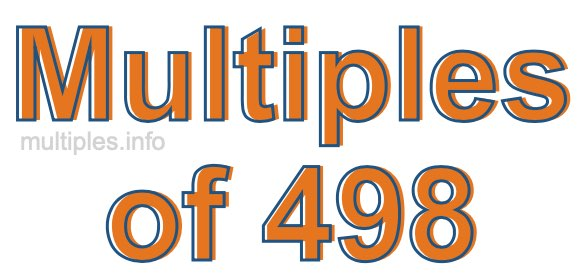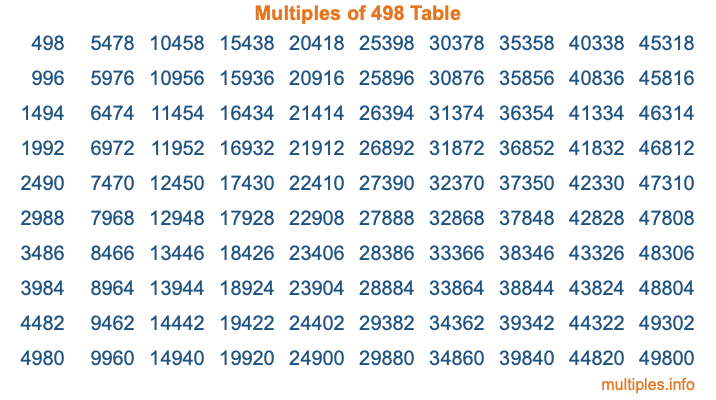Multiples of 498Welcome to the Multiples of 498 page. Here we will first teach you everything you will ever need to know about the multiples of 498, and then give you a study guide summary of everything we taught you to make sure you remember it all. Use this page to look up facts and learn information about the multiples of 498. This page will make you a multiples of four hundred ninety-eight expert!

Definition of Multiples of 498
Multiples of 498 are all the numbers that when divided by 498 equal an integer. Each of the multiples of 498 are called a multiple. A multiple of 498 is created by multiplying 498 by an integer.

Therefore, to create a list of multiples of 498, you start with 1 multiplied by 498, then 2 multiplied by 498, then 3 multiplied by 498, and so on for as long as you want. Thus, the list of the first five multiples of 498 is 498, 996, 1494, 1992, and 2490. To see a larger list of multiples of 498, see the printable image of Multiples of 498 further down on this page. We also have a category where you can choose any nth multiple of 498.

Multiples of 498 Checker
The Multiples of 498 Checker below checks to see if any number of your choice is a multiple of 498. In other words, it checks to see if there is any number (integer) that when multiplied by 498 will equal your number. To do that, we divide your number by 498. If the the quotient is an integer, then your number is a multiple of 498.

Is  a multiple of 498?

Least Common Multiple of 498 and ...
A Least Common Multiple (LCM) is the lowest multiple that two or more numbers have in common. This is also called the smallest common multiple or lowest common multiple and is useful to know when you are adding our subtracting fractions. Enter one or more numbers below (498 is already entered) to find the LCM.

Check out our LCM Calculator if you need more details about the Least Common Multiple or if you need the LCM for different numbers for adding and subtraction fractions.

nth Multiple of 498
As we stated above, 498 is the first multiple of 498, 996 is the second multiple of 498, 1494 is the third multiple of 498, and so on. Enter a number below to find the nth multiple of 498.

th multiple of 498

Multiples of 498 vs Factors of 498
498 is a multiple of 498 and a factor of 498, but that is where the similarities end. All postive multiples of 498 are 498 or greater than 498. All positive factors of 498 are 498 or less than 498.

Below is the beginning list of multiples of 498 and the factors of 498 so you can compare:

Multiples of 498: 498, 996, 1494, 1992, 2490, etc.

Factors of 498: 1, 2, 3, 6, 83, 166, 249, 498

As you can see, the multiples of 498 are all the numbers that you can divide by 498 to get a whole number. The factors of 498, on the other hand, are all the whole numbers that you can multiply by another whole number to get 498.

It's also interesting to note that if a number (x) is a factor of 498, then 498 will also be a multiple of that number (x).

Multiples of 498 vs Divisors of 498
The divisors of 498 are all the integers that 498 can be divided by evenly. Below is a list of the divisors of 498.

Divisors of 498: 1, 2, 3, 6, 83, 166, 249, 498

The interesting thing to note here is that if you take any multiple of 498 and divide it by a divisor of 498, you will see that the quotient is an integer.

Multiples of 498 Table
Below is an image of the first 100 multiples of 498 in a table. The table is in chronological order, column by column. The first column has the first ten multiples of 498, the second column has the next ten multiples of 498, and so on.The Multiples of 498 Table is also referred to as the 498 Times Table or Times Table of 498. You are welcome to print out our table for your studies.

Negative Multiples of 498
Although not often discussed or needed in math, it is worth mentioning that you can make a list of negative multiples of 498 by multiplying 498 by -1, then by -2, then by -3, and so on, to get the following list of negative multiples of 498:

-498, -996, -1494, -1992, -2490, etc.

Multiples of 498 Summary
Below is a summary of important Multiples of 498 facts that we have discussed on this page. To retain the knowledge on this page, we recommend that you read through the summary and explain to yourself or a study partner why they hold true.

There are an infinite number of multiples of 498.

A multiple of 498 divided by 498 will equal a whole number.

498 divided by a factor of 498 equals a divisor of 498.

The nth multiple of 498 is n times 498.

The largest factor of 498 is equal to the first positive multiple of 498.

498 is a multiple of every factor of 498.

498 is a multiple of 498.

A multiple of 498 divided by a divisor of 498 equals an integer.

498 divided by a divisor of 498 equals a factor of 498.

Any integer times 498 will equal a multiple of 498.

Multiples of a Number
Here you can get the multiples of another number, all with the same attention to detail as we did for multiples of 498 on this page.

Multiples of
Multiples of 499
Did you find our page about multiples of four hundred ninety-eight educational? Do you want more knowledge? Check out the multiples of the next number on our list!